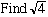Name: Frank Question: In a recent quiz we where asked the square root of four. The quizmaster gave the answer as not only 2 but also -2. Being pedantic I queried this. Although -2 squared is 4, does it follow that the root of 4 is -2 Can you enlighten us? Regards Frank Hi Frank, It depends whether you define "square root of 4" as "the positive number whose square is 4" or "any number whose square is 4". I think that in the question "Find the square root of 4", the first definition is implicit, otherwise you should have been asked "Find all square roots of 4". If the instructions werethen the answer is 2. The surd symbol, applied to a non-negative real number x, always returns the non-negative real number y that satisfies y 2 = x. Claude and Penny Go to Math Central